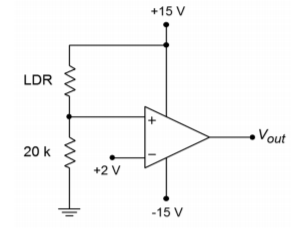Skip to main content

# 2.6: Problems

•• Contributed by James M. Fiore
• Professor (Electrical Engineering Technology) at Mohawk Valley Community College

## Review Questions

1. What is an op amp?

2. Give several examples of where op amps might be used.

3. What is the typical stage layout of an op amp?

4. What comprises the first stage of a typical op amp?

5. What comprises the final stage of a typical op amp?

6. How does integrated circuit design differ from discrete design?

7. What is a comparator, and how might it be used?

8. What is meant by monolithic planar construction?

9. What is a mask, and how is it used in the construction of IC op amps?

10. What is the process of diffusion and how does it relate to the construction of IC op amps?

11. What are the advantages of monolithic IC construction?

12. How does hybrid construction differ from monolithic construction?

## Problems

1. For the circuit in Figure $$\PageIndex{1}$$, find $$V_{out}$$ for the following inputs:

a) 0 V

b) -1 V

c) +2 VFigure $$\PageIndex{1}$$

2. For the circuit in Figure $$\PageIndex{2}$$, find $$V_{out}$$ for the following inputs:

a) 0 V

b) -4 V

c) +5 V

d) -0.5 VFigure $$\PageIndex{2}$$

3. For the circuit in Figure $$\PageIndex{3}$$, find $$V_{out}$$ for the following inputs:

a) 0 V

b) -2 V

c) +1 V

d) -0.5 VFigure $$\PageIndex{3}$$

4. Sketch $$V_{out}$$ if $$V_{in}(t) = 1 \sin 60t$$ in Figure $$\PageIndex{1}$$.

5. Sketch $$V_{out}$$ if $$V_{in}(t) = 2 \sin 20t$$ in Figure $$\PageIndex{2}$$.

6. Sketch $$V_{out}$$ if $$V_{in}(t) = 3 \sin 10t$$ in Figure $$\PageIndex{3}$$.

7. A Temperature Dependent Resistor is used in the comparator of Figure $$\PageIndex{4}$$. At what temperature will the comparator change state?Figure $$\PageIndex{4}$$

8. What is the value of the Light Dependent Resistor at the comparator trip point in Figure $$\PageIndex{5}$$?Figure $$\PageIndex{5}$$

9. What reference voltage is required for a $$50^{\circ}$$C trip point in Figure $$\PageIndex{4}$$?Figure $$\PageIndex{6}$$

10. A thermal fuse is device found in such common items as coffee makers. Normally, its resistance is very low, ideally 0 $$\Omega$$. With the application of excessive heat, the fuse opens, presenting a very high resistance, ideally infinite. Explain the operation of the circuit in Figure $$\PageIndex{6}$$. Is the output voltage normally high or low?

11. A strain gauge is a device that can be used to measure the amount of bend or deflection in a part that it is attached to. It can be connected so that its resistance rises as the bend increases. What resistance is required to trip the comparator of Figure $$\PageIndex{7}$$?Figure $$\PageIndex{7}$$

12. Determine the current flowing out of the collector of $$Q_{13}$$ in Figure 2.2.4.

## Computer Simulation Problem

13. Alter the simple simulation model presented in the chapter to include saturation effects. (Hint: Consider a device that limits voltage.)